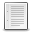File : Details

# Modeling samples with xCos

Tutorials samples in xCos modeling for beginers
Details
Version
1.0
Author
Sahib Huseynov
Maintainer
Sahib Huseynov
Category
Supported Scilab Version
Creation Date
September 8, 2015Description
```            Here are presented several simple problems on mathematical modeling in ecology.
I think that it can be useful for beginners in Xcos.
1)Simple ecological model: dx/dt=kx.
2) Verhilst ecological model: dx/dt=ax-bx^2
3) The Volterra-Lotka ecological model: dx/dt=ax+bx^2+cxy, dy/dt=ky+mxy.
Where, x and y population number, t - time; a, b and k constant coefficients of
an ecosystems.            ```Files (1)macosx binary for Scilab .x
```	Here are presented several simple problems on mathematical modeling in ecology.
I think that it can be useful for beginners in Xcos.
1)Simple ecological model: dx/dt=kx.
2) Verhilst ecological model: dx/dt=ax-bx^2
3) The Volterra-Lotka ecological model: dx/dt=ax+bx^2+cxy, dy/dt=ky+mxy.
Where, x and y population number, t - time; a, b and k constant coefficients of
an ecosystems.
```News (0)Comments (0)